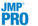Publication date: 07/30/2020

##Structural Equation Model Fit Report

Each time you click Run, a Structural Equation Model report for the specified model appears. By default, this report contains a Summary of Fit report, a Parameter Estimates report, and a Path Diagram.

Summary of Fit

Table of information about the model fit, including any convergence issues. The following statistics are reported in this table:

Sample Size

The number of observations (rows) used to fit the model.

Rows with Missing

The number of observations (rows) that contained at least one missing value. All missing values are handled using full information maximum likelihood (Finkbeiner 1979).

Iterations

The number of iterations used to fit the model.

-2 Log Likelihood

The log-likelihood of the fitted model multiplied by -2. This value can be used to compare nested models; the difference between two models’ -2 Log Likelihood values is chi-square distributed with degrees of freedom equal to the difference of degrees of freedom between the models.

Number of Parameters

The number of freely estimated parameters in the model.

AICc

The corrected Akaike information criterion. This value can be used to compare models, where a smaller number indicates a better model fit. See Statistical Details for the Structural Equation Models Platform.

BIC

The Bayesian information criterion. This value can be used to compare models, where a smaller number indicates a better model fit. See Statistical Details for the Structural Equation Models Platform.

ChiSquare

The chi-square statistic for the model.

DF

The degrees of freedom for the chi-square test for model fit.

Prob>ChiSq

The p-value of the chi-square statistic for the model.

CFI

The Bentler’s comparative fit index (CFI), which provides additional guidance for determining model fit. The CFI is bounded between 0 and 1. Values greater than 0.90 are preferred (Browne and Cudeck 1993; Hu and Bentler 1999). See Statistical Details for the Structural Equation Models Platform.

RMSEA

The root mean square error of approximation (RMSEA), which provides additional guidance for determining model fit. The RMSEA is bounded between 0 and 1. Values less than 0.10 are preferred (Browne and Cudeck 1993; Hu and Bentler 1999). See Statistical Details for the Structural Equation Models Platform.

Lower 90%

The 90% lower confidence limit for the RMSEA. See Statistical Details for the Structural Equation Models Platform.

Upper 90%

The 90% upper confidence limit for the RMSEA. See Statistical Details for the Structural Equation Models Platform.

Parameter Estimates

Table of estimates for the model parameters. The table is organized in sections for Means/Intercepts, Loadings, Regressions, and Variances. For each estimate, a standard error (Std Error), Wald test statistic (Wald Z), and a corresponding p-value (Prob>|Z|) are given.

Path Diagram

Shows the path diagram representation of the fitted model. See Diagram Tab.